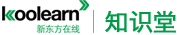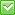<%/*%> <%*/%>热门：已解决问题
math

<div>Q 28
For any positive integer n, the sum of the first n positive integers equals [n(n+1)]/2. What is the sum of all the even integers between 99 and 301?
A. 10100   B.20200   C.22650    D40200   E.45150

all the even integers between 99 and 301: (301-99)/2=101
the sum of these: [101(101+1)]/2

Q37
A jar contains 16 marbles, of which 4 are red, 3 are blue, and the rest are yellow. If 2 marbles are to be selected at random from the jar, one at a time without being replaced, what is the probability that the first marble selected will be red and the second marble selected will be blue?
A. 3/64   B.1/20   C.1/16   D.1/12   E.1/8
<div style="margin: 0in 0in 0pt"> </div>
</div>最佳答案

28:Here first term is a=100
Last term=300=a+(n-1)d where n=no of terms and d=common difference=2 (even nos)
so n =(300-100)/2 + 1 = 101
Now you have everything.
Put them in the formula of Sum of an AP =(n/2)[2a+(n-1)d]

Remember this is the general formula for sum of an AP. When you take say first n integers, where common difference =1 and 1st term=1, then the above formula deduce to (n/2)[2+(n-1)*1] = n(n+1)/2

37:I agree B. 4/16 Red = 1/4. You are not replacing so the prob. of blue is 3/15.

1/4 * 1/5=1/20